# Forecasting - Metrics for Time Series Forecasts - EdsCave

Go to content

## Forecasting - Metrics for Time Series Forecasts

Forecasting

If you're engaged in the task of making time-series forecasts, their accuracy is something you are probably concerned about. And if for whatever reason you aren't all that concerned, then other people, the  customers for your forecasts -  purchasing, sales, and senior management - probably are.  Since time-series forecasts involve numbers, there are numerous ways of quantitatively evaluating their quality.  This article will describe a few of the more common.

A time-series forecast requires making a quantitative prediction of some future set of values for some quantitative recurring sequence. For example, a manufacturer of breakfast cereal will keep historical records of how many boxes of a given cereal consumers have bought each month.  Knowing how many boxes to manufacture next month is important for two reasons. First, if you make too many, they will sit in inventory and go stale – something that eventually happens no matter how many preservatives are added. The other alternative is the dreaded stockout – where you don't make enough product, the stores sell out of your product and leave disappointed consumers who go on to buy a competitor's product instead.  Being able to provide accurate forecasts – neither too high nor too low – serves a genuine business need.  While forecasting metrics won't make your forecast for you, they can be useful in telling you how you are doing as forecaster and helping you determine which particular forecasting methods work best in your situation.

Defining Error

The fundamental core of any evaluation technique is the definition of deviation from the ideal or error.  For the purposes of comparing our forecasts to reality, we will define error as the difference between the actual value for a given actual datum in the time series (Ai) and the forecast value (Fi):The order of the subtraction in this definition of error may be a little counter-intuitive, as a predicted data point that is higher than the actual value yields a negative error value. I am using this definition as it is a common convention in forecasting literature (and incidentally, also common in the machine learning world as well).

Absolute Error Metrics

While being able to quantify the error for each data point is important,  basing an evaluation off a single data point is neither particular wise nor useful.  Because the actual data will typically contain some 'random' variation (or at least poorly understood variation) and some of that noise will find its way into any forecast based on it, some portion of the Actual-Forecast error term will be noise. For this reason you will find that in a given series of predictions, some will be better (and some worse) than others. Properly evaluating a forecast's accuracy requires the consideration of numerous predictions, preferably lots of predictions.

Consider the hypothetical monthly forecast and actual sales for ChocoNuclear Crunch, shown in the table below.  The actual figures are what would be counted shipping from the factory at the end of each month, while the forecast figures would normally be developed before this time, when the actual were not known. It is for this reason that while there is a forecast figure for October, the 'actual' figure is labeled TBD, because the month's data is not available.  The final line in the table is the 'Ai – Fi' error for each month.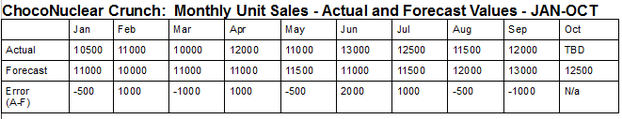The first metric we will look at is the Mean Error (ME), which is simply the average of all the individual errors and given by the formula below.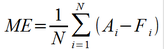Mean Error measures a forecast's bias, which is one type of error.  If your forecasts are consistently low, the ME metric will be positive, while if they run consistently high, the ME metric will be negative. When applied to the above table of ChocoNuclear Crunch sales, this metric yields 166.7, indicating that the average forecast is biased a little lower than the actual shipments.  Interestingly, if you were to have a forecast where  some individual errors were positive, and others negative, the overall ME could equal zero even with a set of forecasts that widely diverged from actual. For this reason, while ME can indicate systematic bias (valuable information), it is not useful as a measure of how 'good' the forecast is.

In statistics, it is very common to 'square' error terms. This avoids the pitfall where positive and negative errors can cancel each other out (as was the case with ME), as a squaring operation makes all error terms positive. This same technique can be readily applied to time-series errors. The formula for Mean Squared Error (MSE) is shown below.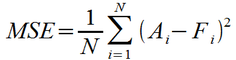One drawback of the squaring operation makes the magnitude of MSE not directly comparable to that of the original errors – for example,  the MSE for the ChocoNuclear Crunch forecast is 1,083,333, which is difficult to relate to the magnitudes of the monthly shipments. One solution is to take the square root, yielding the metric Root-Mean Square Error (RMSE) metric, as shown below.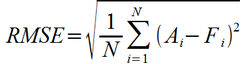The RMSE metric for ChocoNuclear Crunch yields 1040, which is of comparable magnitude to the individual monthly errors. With MSE, as with the other metrics to be described in this article, a lower value indicates a better forecast. One property of the RMSE metric is that it more heavily penalizes larger errors due to the squaring operation. For example, an error of '2' in a given month carries as much weight as an error of '1' in four separate months combined.   This feature has both advantages and disadvantages. On the positive side, it means that the RMSE metric will favor a forecasting technique in which the individual errors tend to be of consistent magnitude, as wide variations in error will tend to drive the RMSE metric higher.  The disadvantage is the flip side of this – a few bad forecast points in an otherwise very good forecast can drive up the RMSE figure, masking otherwise good performance.

One way to minimize the effects of a few 'outlier' bad forecast points is to switch from squaring the error to taking the absolute value.  In the Mean Absolute Error (MAE) metric shown below, large errors only have a proportional effect on the overall metric.  An error of '2' makes the same contribution as two errors of '1'.Relative Error Metrics

While the absolute error metrics presented above are fine for comparing the effectiveness of different forecasting techniques when applied to the same time series, they are less useful when comparing effectiveness across multiple time-series, especially those of different magnitude.  For example,  if a given forecast showed an MAE of 27 units for a product selling 100,000 units/month, in many quarters that would be considered stunningly on-target. On the other hand, the same MAE for a product selling 50 units/month would likely be considered very, very poor.

One way to make a metric that is useful for comparing forecasts on disparate-magnitude time series is to use relative percentage error.  This is obtained by dividing the individual forecast point error by the actual value, as shown below, and multiplying by 100 to scale to percent.  This scales the error by the magnitude of the actuals.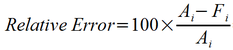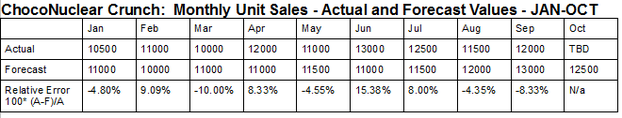The relative error then may be substituted for the error terms in the metrics described above. The most commonly encountered relative error metrics are Mean Percentage Error (MPE), and Mean Absolute Percentage Error (MAPE).  For the above forecast, MPE = 0.98%, while MAPE = 8.09%. As was the case for ME,  MPE is a metric for high or low bias, while MAPE is useful as an overall accuracy. The formulas for MEA and MAPE are shown below.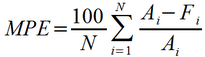While it is possible to develop a Root-Mean Square Percentage Error (RMSPE), analogous to the RMSE metric, this is not as commonly seen as MAPE.

Unlike the absolute error metrics initially presented, all of the relative error metrics suffer from the limitation that they can't be used on forecast data which have '0' values for any of the actuals, as this results in division-by-zero, yielding undefined results.  Related to this is the problem of what happens when the actual value is merely very small compared to a forecast.  While the individual error terms have a downside limit of 0, there is no corresponding upside limit, so actual values close to zero can have a disproportionate effect on the accuracy reported by these metrics.  These issues  can seriously limit the effective use of these metrics for systems with widely varying actual values or systems with sporadic demand (and consequently actual data points of '0').

The article has discussed a couple of common error metrics used for evaluating the quality of time-series forecasts. The table below provides a summary.15 DEC 2016